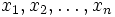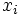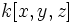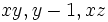# Permutation of regular sequence is not necessarily regular

## Statement

Let$R$ be a commutative unital ring,$M$ a$R$-module, and$x_1,x_2,\ldots,x_n$ a regular sequence in$R$, for the module$M$. Then, it is not necessarily true that every permutation of the$x_i$s is regular.

## Partial truth

The following are true:

• If$R$ is a Noetherian local ring, and the regular sequence comprises elements in the unique maximal ideal, then any permutation of it is regular.
• If$R$ is a graded ring, and all the elements in the regular sequence are homogeneous elements, then every permutation is regular.
• Any permutation of a regular sequence of length one is regular (obviously)
• For a unique factorization domain, and when the module is the ring itself, a sequence of length two is regular, if and only if the two elements are relatively prime. Thus, any permutation of a regular sequence of length two is regular.
• For a principal ideal domain, when the module is the ring itself, there cannot exist regular sequences of length more than two, so any permutation of a regular sequence is regular.

## Example

One example uses the polynomial ring$k[x,y,z]$ over a field. Consider the sequences:$xy, xz, y - 1$

versus:$xy, y - 1, xz$

The first one of these is regular, while the second clearly isn't: in the second sequence, the term$xz$ is actually equal to zero modulo the first two terms.